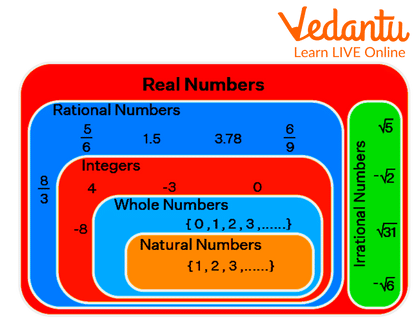Courses
Courses for Kids
Free study material
Free LIVE classes
More

# Real NumbersLIVE
Join Vedantu’s FREE Mastercalss

## What do you understand about Real Numbers?

Any number that may be found in the real world is a real number. We are constantly surrounded by numbers. Integers are used to measure temperature, rational numbers are used to represent fractions, irrational numbers are used to find the square root of a number, etc. To count objects, we utilise natural numbers. These several types of numbers combine to form a collection of real numbers.

### Real Number

Any number that can be found in the real world is a real number. We are constantly surrounded by numbers. Temperature is measured using integers, fractions are represented using rational numbers, square roots are calculated using irrational numbers, etc. Natural numbers are used to count objects. These various types of numbers combine to form a collection of real numbers.Real Numbers

### Whole Numbers

All natural numbers and 0 are included in the category of whole numbers. They are a subset of real numbers, which exclude negative numbers, decimals, and fractions. Whole numbers include counting numbers as well.

### Natural Numbers

All positive numbers from 1 to infinity are considered natural numbers and are therefore a part of the number system. Because they don't contain zero or negative numbers, natural numbers are also known as counting numbers.

## Types of Real Numbers

We are aware that real numbers can be both irrational and rational. As a result, there is no real number that falls into either the rational or irrational category. Simply put, it means that each number we choose from R is either rational or irrational. Simply put, it means that each number we choose from R is either rational or irrational.

### Rational Number

Any number that can be expressed as a fraction p/q is referred to as a rational number. The fraction has a numerator of "p" and a denominator of "q," where "q" is not equal to zero. Natural numbers, whole numbers, decimals, and integers can all be rational numbers.

### Irrational Numbers:

The groups of real numbers known as irrational numbers are those that cannot be written as a fraction of the form p/q, where p and q are integers and q is not equal to zero, (Pi) for instance, is an irrational number. The decimal value, in this case, is 3.14159265…., which never ends.

### Real Numbers Between 2 and 7

The collection of both rational and irrational numbers is known as a real number. In light of the question, we can write 3 because it is a natural number if we are asked to write a real number between 2 and 7.

## Conclusion

In Mathematics, rational numbers and irrational numbers are together obtained from a set of real numbers. The set of real numbers is represented by the letter R. Therefore, it indicates that every real number is either a rational number or an irrational number. In either case, it contains a non–terminating decimal depiction. In the instance of rational numbers, the decimal depiction is repeating (including repeating zeroes) and if the decimal depiction is non–repeating, it is an irrational number.

## Solved Examples

Example 1: Find Real Numbers between 2 and 7.

Solution: The collection of both rational and irrational numbers is known as a real number. In light of the question, we can write 3 because it is a natural number if we are requested to write a real number between 2 and 7 as while counting numbers 3 comes in between 2 and 7.

Example 2: Give any 3 real numbers between 20 and 40.

Solution: We will count numbers from 20 to 40 and all those numbers which come between 20 to 40 will be real. For instance, 23, 31, and 39 are the 3 real numbers between 20 to 40.

Example 3: Using real numbers, say whether something is true or false.

a) Real numbers exist for every irrational number.

b) Each and every real number is a rational number.

c) Each and every real number is a complex number.

Solution:

a) Every irrational number is a real number, it is true.

b) It is accurate to say that each rational number is a real number.

c) Complex false numbers are not actual numbers.

Example 4: Find two Irrational numbers between 2 and 3.

Solution: The real number which can be expressed as a ratio of integers is rational; for example, 2 is rational.

Similarly, an irrational number appears as a fractional increment that is neither ending nor recurrent.

Find irrational numbers between 2 and 3:

As a result, the number of irrational numbers between 2 and 3 and $\sqrt 5,\sqrt 6 and \sqrt 7$ whose perfect square roots do not exist.

Hence, the three irrational numbers between 2 and 3 are “$\sqrt 5,\sqrt 6 and \sqrt 7$ ”.

Last updated date: 02nd Oct 2023
Total views: 91.8k
Views today: 2.91k

## FAQs on Real Numbers

1. Is zero a real number?

Ans: Real numbers include the number zero and can be either positive or negative. Because they are not imaginary, which is a different type of number system, they are referred to as real numbers.

2. Is a complex number's subset the real number?

Ans: Yes, since a complex number is made up of both real and fictitious numbers. Therefore, real and imaginary numbers are subsets of the complex number if it is a set.

3. Is zero a real number?

Ans: Because it is a member of the set of whole numbers, which is a subset of real numbers, zero is a real number.